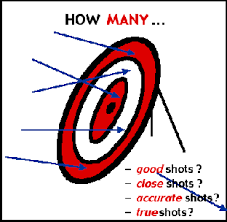# Maths is a little without Sets!

Probability Level 2$\large\color{darkred}{B=\{ \{ M,A,T,H,S \} \}}$

Find the cardinal number of the set $\color{darkred}{B}$.

Note: The cardinal number of a set is equal to the number of elements contained in the set.

×